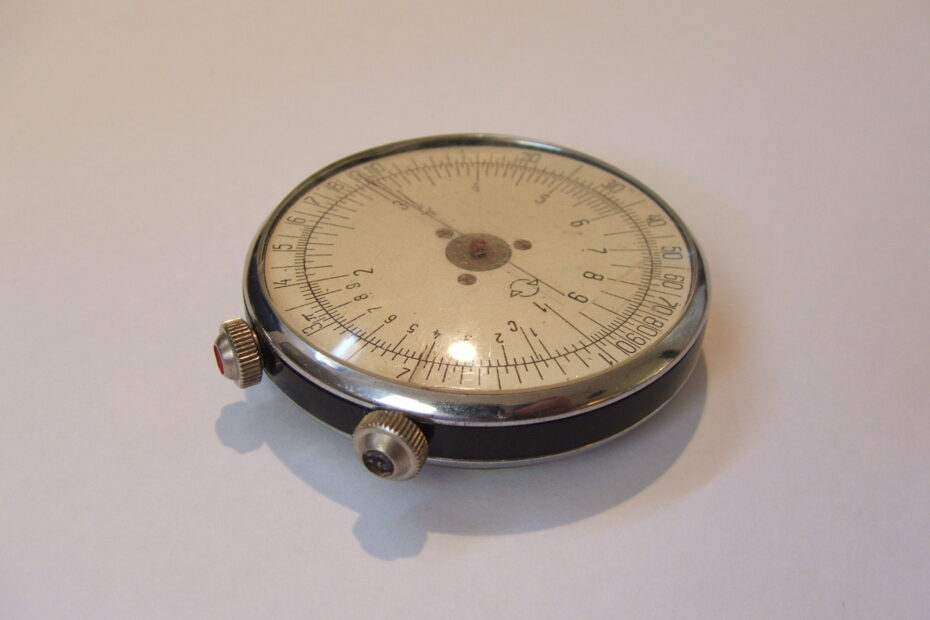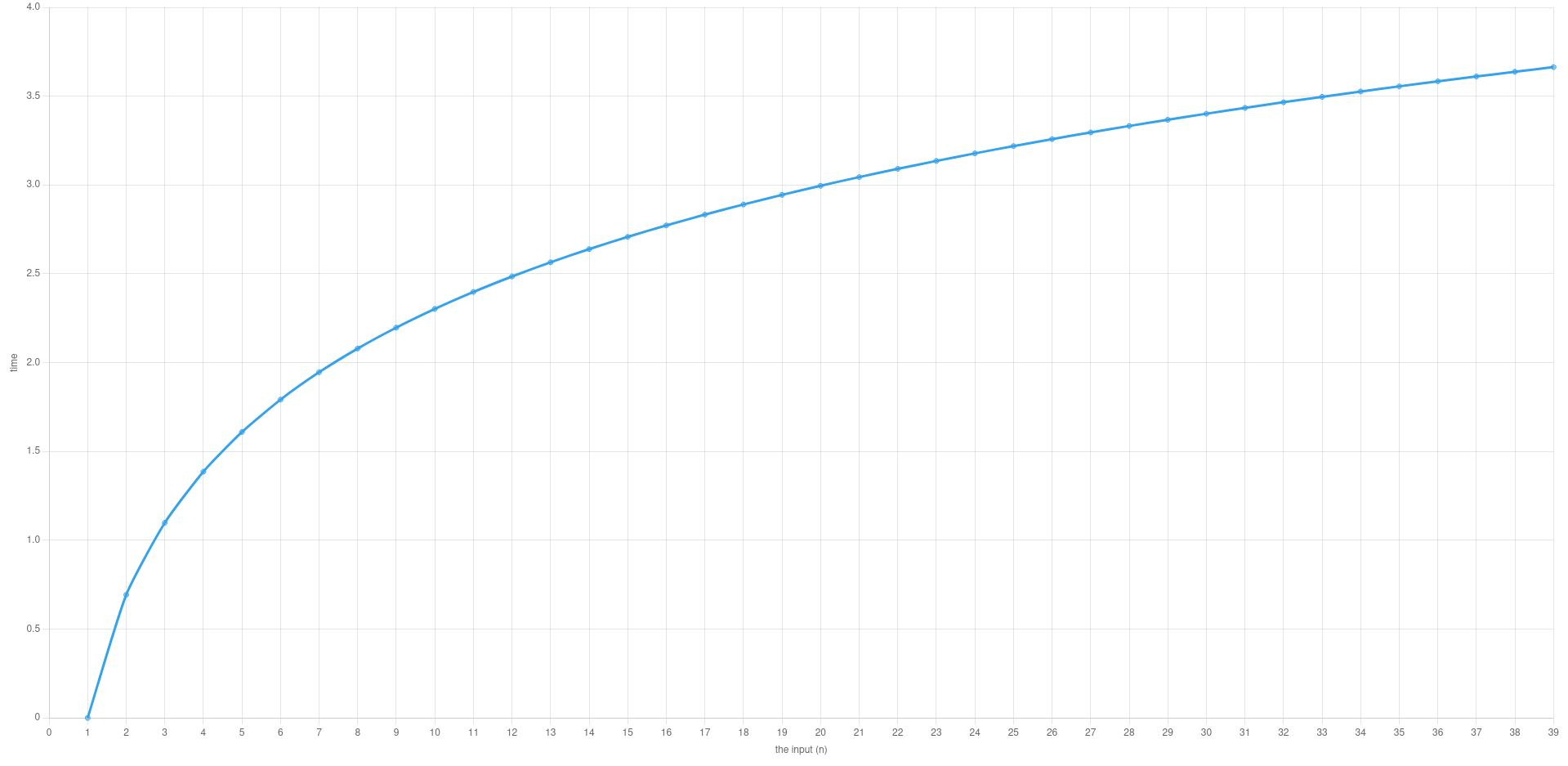# O(log N) Algorithm Example### Common Algorithm Time Complexities

When dealing with algorithms, it is important to consider their time complexity. The time complexity of an algorithm refers to how its performance scales with the size of the input. Let’s see the most common algorithm time complexities in order from the fastest to the slowest:

• O(1) – constant time complexity. The algorithm takes the same amount of time to run, regardless of the size of the input.
• O(log N) – logarithmic time complexity. The time required to run the algorithm increases logarithmically with the size of the input.
• O(N) – linear time complexity. The time required to run the algorithm increases linearly with the size of the input.
• O(N log N) – linearithmic time complexity. The time required to run the algorithm increases linearly with the size of the input, multiplied by the logarithm of the input size.
• O(N^2) – quadratic time complexity. The time required to run the algorithm increases quadratically with the size of the input.
• O(N^3) – cubic time complexity. The time required to run the algorithm increases cubically with the size of the input.
• O(2^N) – exponential time complexity. The time required to run the algorithm increases exponentially with the size of the input.
• O(N!) – factorial time complexity. The time required to run the algorithm increases factorially with the size of the input.

The actual running time of an algorithm can vary depending on the specific hardware and software used. Therefore, time complexity should be used as a theoretical measure of performance, rather than an absolute measure.

### What is O(log N) Time Complexity?

O(log n) time complexity refers to an algorithm that takes time proportional to the logarithm of the input size, as the size of the input increases. More specifically, it means that the time required to solve the problem using this algorithm increases slowly as the size of the input increases.The base of the logarithm is not important for big O notation, so O(log n) represents any logarithmic base, such as base 2 or base 10. An algorithm with O(log n) time complexity is generally considered efficient and fast, especially when compared to algorithms with higher time complexities like O(N) or O(N^2).

The most common example of an algorithm with O(log n) time complexity is the binary search algorithm, which can find a specific value in a sorted list of n items by successively dividing the list into half at each iteration. Other examples of O(log n) algorithms include various divide-and-conquer algorithms, such as the QuickSort algorithm and the MergeSort algorithm.

O(log n) time complexity is considered a desirable time complexity in many cases because it allows us to solve problems efficiently even when the input size is very large. In this article, we will discuss an example of an algorithm with this time complexity: finding the greatest common divisor (GCD) of two positive integers. Did you know that we offer a unique online course that boosts your C# career? Check it out here!

### O(log N) Example: (GCD) Euclidean Algorithm

The greatest common divisor (GCD) of two positive integers `a` and `b` is the largest positive integer that divides both `a` and `b` without leaving a remainder. There are many methods to find the GCD of two integers, but one of the most efficient ones is the Euclidean algorithm. The Euclidean algorithm is a simple and recursive algorithm that relies on the fact that the GCD of two numbers is the same as the GCD of the smaller number and the remainder of the larger number divided by the smaller number.

#### Steps

Let’s take a closer look at the steps of the Euclidean algorithm:

1. Ensure that `a` is greater than `b`. If `b` is greater than `a`, swap the values of `a` and `b`.
2. Compute the remainder `r` of `a` divided by `b`.
3. If `r` is equal to `0`, then the GCD of `a` and `b` is `b`. Otherwise, set `a` to `b` and `b` to `r`, and go back to step 2.

The Euclidean algorithm has a time complexity of O(log N), where N is the larger of the two input integers. This is because, in each iteration of the algorithm, the size of the inputs is reduced by a factor of at least 2, which means that the number of iterations required is proportional to log N.

### C# Implementation

Here’s an example implementation of the Euclidean algorithm in C#:

To use this function, you can call it with two integers as arguments, like this:

And the output is:

In this example, the function takes two integers as input and then uses the Euclidean algorithm to compute the GCD of these two integers. The function returns the GCD as an integer.

### Other Algorithms With the O(log N) Time Complexity

Let’s see some examples of algorithms with a time complexity of O(log N).

• Binary search: This is a search algorithm that works by repeatedly dividing a sorted array in half until the target element is found, or until it is clear that the target element is not in the array. The time complexity of binary search is O(log N).
• Finding the exponentiation: Given a base and an exponent, the algorithm computes the result by repeatedly squaring the base and dividing the exponent by two until the exponent is zero. The time complexity of this algorithm is also O(log N).
• Balanced binary search tree operations: Insertion, deletion, and search operations in balanced binary search trees, such as AVL trees and Red-Black trees, all have a time complexity of O(log N).
• Finding the greatest common divisor (GCD): Given two positive integers a and b, the algorithm computes the GCD by repeatedly taking the remainder of the larger number divided by the smaller number, until the smaller number is zero. The time complexity of this algorithm is also O(log N).
• Finding the minimum/maximum element in a heap: Heaps are data structures that maintain the property that the root node has either the minimum or maximum value in the tree. The time complexity of finding the minimum or maximum element in a heap is O(log N).

• Efficient: Algorithms with O(log N) time complexity are generally very efficient and can solve problems quickly, even as the input size grows larger.
• Scalable: Logarithmic algorithms are highly scalable, meaning that they can handle large input sizes with ease.

• Limited use cases: Not all problems can be solved using logarithmic algorithms. In some cases, algorithms with higher time complexities like O(N) or O(N^2) may be necessary.
• Limited flexibility: Logarithmic algorithms often require the input to be sorted or meet other specific requirements, which can limit their flexibility in certain situations.
• High implementation complexity: Some logarithmic algorithms can be difficult to implement, requiring a deep understanding of mathematical concepts like logarithms and recursive functions.